# How to Extract Last Word in Microsoft Excel

If you are required to extract last word from a string in excel, then you can use combination of various functions. In this article, we will focus on extracting last word & last occurrence of specific character from a cell.

Question: How can I extract the last word as the data I have contains separator as “space”, “comma”, “dash” or any other thing.

Following is the snapshot of preferred output data: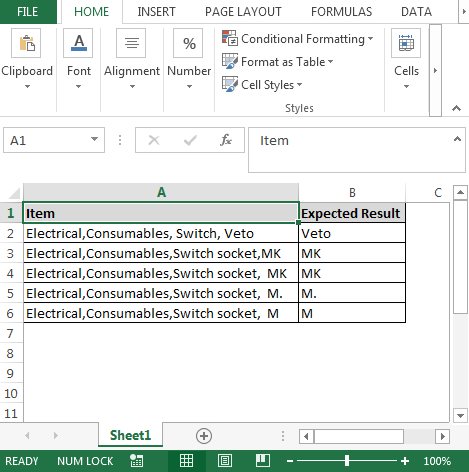We will find the same solution with different formulas.

Let us first find the solution using array.

• This formula has the combination of TRIM, RIGHT, LEN, MAX, ROW, INDIRECT & MID functions
• In cell C2, the formula is
• {=TRIM(RIGHT(A2,LEN(A2)-MAX(ROW(INDIRECT("1:"&LEN(A2)))*(MID(A2,ROW(INDIRECT("1:"&LEN(A2))),1)=","))))}
• Copy the formula to get the desired output

Note: this is an array formula; therefore, use CTRL + SHIFT + ENTER keys together.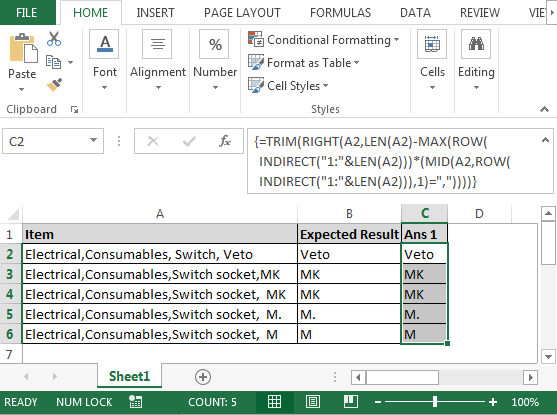Let us see the second solution.

• This formula has the combination of TRIM, SUBSTITUTE, RIGHT &REPT functions
• In cell D2, the formula is
• =TRIM(SUBSTITUTE(RIGHT(SUBSTITUTE(A2,",",REPT(",",LEN(A2))),LEN(A2)),",",""))
• Copy the formula to get the desired output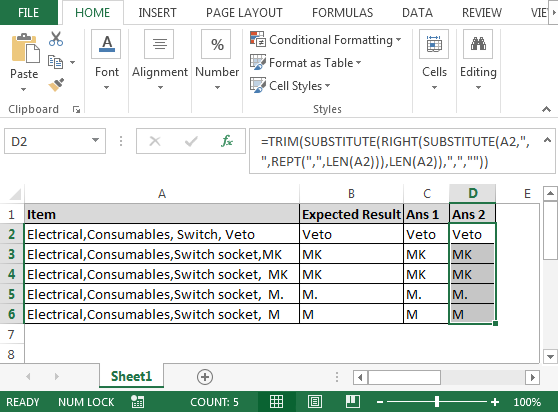Let us see the third solution.

• This formula has the combination of TRIM, RIGHT, SUBSTITUTE & REPT functions
• In cell E2, the formula is
• =TRIM(RIGHT(SUBSTITUTE(SUBSTITUTE(A2,","," ")," ",REPT(" ",256)),256))
• Copy the formula to get the desired output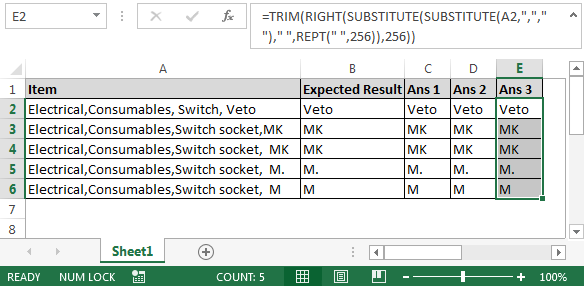Let us see the fourth solution.

• This formula has the combination of TRIM, RIGHT, SUBSTITUTE & REPT functions
• In cell F2, the formula is
• =TRIM(RIGHT(SUBSTITUTE(A2,",",REPT(" ",250)),250))
• Copy the formula to get the desired output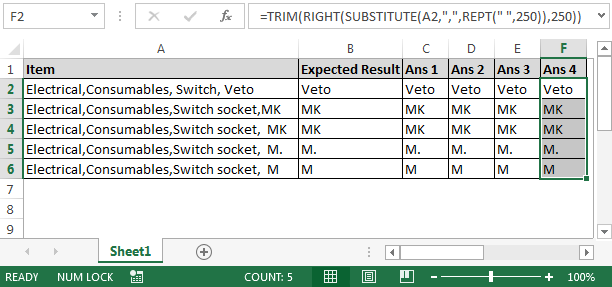Let us see the fifth solution.

• This formula has the combination of TRIM, RIGHT, LEN, FIND & SUBSTITUTE functions
• In cell G2 the formula is
• =TRIM(RIGHT(A2,LEN(A2)-FIND("|",SUBSTITUTE(A2,",","|",3))))
• Copy the formula to get the desired output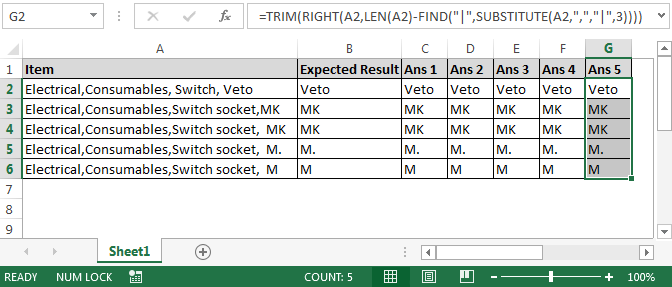In this way, we can use the formula we are comfortable with & get the solution.

Let us take another example in which it is required to find the last occurrence of a specific character.

Question: How can I know the position of the last occurrence of any special character say dot, comma, back slash, etc.

Following is the snapshot of the column A that has multiple “\” & the formula needed to find the position of the last occurrence of back slash (\)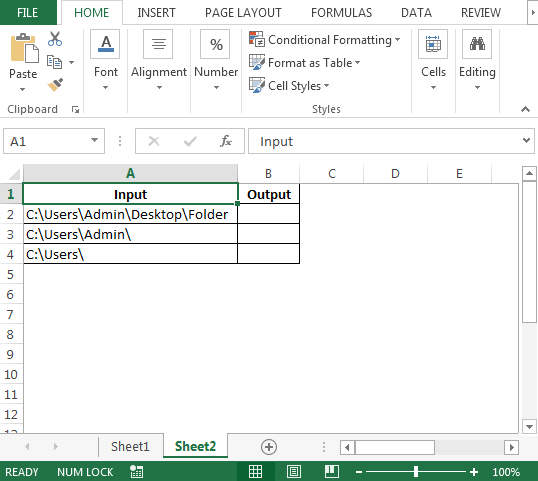• To get the position of the last \, you would use this formula in cell B2:
• =FIND("@",SUBSTITUTE(A2,"\","@",(LEN(A2)-LEN(SUBSTITUTE(A2,"\","")))/LEN("\")))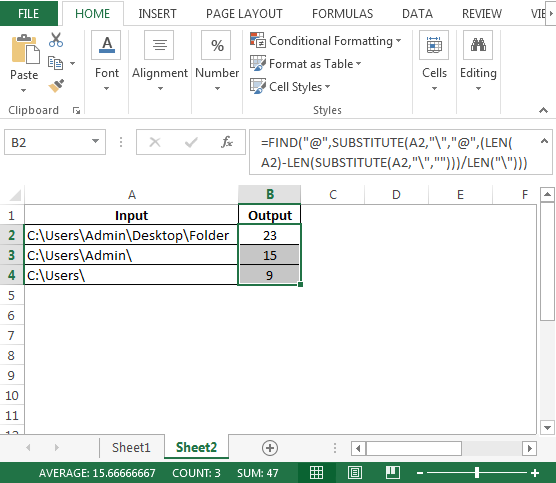Formula explanation:

• The above formula tells us the right-most “\” is at character/position 23, 15 & 9.
• We use find for "@" and substitute the very last "\" with "@". It determines the last one by using
• (len(string)-len(substitute(string, substring, "")))\len(substring)

In the above scenario, the substring is basically "\" which has a length of 1, so we can leave at the end and use the following formula:

• =FIND("@",SUBSTITUTE(A1,"\","@",LEN(A1)-LEN(SUBSTITUTE(A1,"\",""))))

Conclusion: In this way, we can extract last word & last occurrence of the specific character.